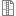# Python implementations

What is an orthogonality space?

Roughly speaking, an orthogonality space is same as an undirected graph where adjacents and (maximal) cliques correspond to the orthogonal elements and (maximal) orthogonal subsets, respectively. We denote an orthogonality space by (X,⊥).

The catalogue of orthogonality spaces

From this point of view, to deal with finite orthogonality spaces, we use our graph database with up to eleven elements. These lists are generated from the C program "nauty and traces" written by Brendan McKay and Adolfo Piperno. We first encrypted their .g6 format and converted them to a user-friendly and readable format for our Python algorithms.

Our catalog of orthogonality spaces can be accessible here (to avoid big size of data, we didn't put eleven element orthogonality spaces to the folder).

What is a linear orthogonality space?

A linear orthogonality space is an orthogonality space such that satisfying two additinonal conditions, say L1 and L2. Explicitly,

(L1) says that the collection of elements orthogonal to distinct elements e and f can be specified in such a way that f is replaced with an element orthogonal to e. (L1) can be seen as a version of orthomodularity; indeed, this property is among its consequences. But more is true; also atomisticity follows and thus (L1) can be regarded as the key property for the representability of X as a linear space.

(L2) can be regarded as a statement complementary to (L1). Indeed, (L2) says that the collection of elements orthogonal to orthogonal elements e and g can be specified in such a way that g is replaced with a third element.

Importance of L1 condition

Looking from the viewpoint of quantum logic and orthomodular structures, here we give our attention to the first condition of linear orthogonality spaces based on the following property:

Thm: Let (X,⊥) fulfills (L1) and let m be the rank of X. Then the set of all closed subsets of X is an atomistic, modular ortholattice of length m.

For more details on linear orthogonality spaces, we refer to the following references:
 T. Vetterlein, Gradual transitivity in orthogonality spaces of finite rank
 J. Paseka and T. Vetterlein, Categories of orthogonality spaces
 K. Emir, D. Kruml, J. Paseka and T. Vetterlein, Linear orthogonality spaces as a new approach to quantum logic

Obtaining all orthogonality spaces fulfilling L1 and/or L2

To obtain all possible L1 or L2 orthogonality spaces, we provide the Python files below. The algorithm reads the orthogonality spaces from the catalog and write the ones in a new file which satisfies the condition L1 or L2 based on your choice.

How to use: In the beginning of the codes, you will see the lines, something like:

source = open("xxx","r")
target = open("yyy", "w")
element_number = z

The only thing you need to do is, for instance, if you want to obtain L1/L2 spaces with an 8 element, relabel xxx = graph8.txt and the arbitrary output file, say yyy = graph8_L1.txt. And replace z with 8. Then execute the program, and that is all. You will see all orthogonality spaces with the condition you requsted in the generated txt file.

Remark: Avoid that L1 and L2 conditions together does not make any sense in finite case. For the reason, see .

Some outcomes of our computer implementation?

Although we are only able to check the orthogonality spaces with a limited number of elements, even these finite results gave us very nice ideas to predict some generalizations:

- For instance, when we checked L1 and L2 together (i.e. linearity), we noticed that there is only one type of linear orthogonality space up to isomorphism - which is exactly the one given as an example in [Categories of orthogonality spaces] and unconnected in the sense of graph theory. Inspiring from these finite results, in  we able to prove that any linear orthogonality space with rank two can be written uniquely up to isomorphism.

- Moreover, we noticed that L1 orthogonality spaces with rank three look similar in our examples. Basically, they are all triangles connected with a common fixed point. Then, inspired from this limited examples, in , we prove that any L1 space with rank three can be written uniquely again.

- As a result, we now can say that, there is no finite linear orthogonality space with rank more than two.

- Catalogue of all L1 spaces with their graphical visualisation can be found here. Here we represent any orthogonality space by means of their maximal orthogonal subsets which are given with square brackets.

AttachmentSizePython source files for L1 and L2 spaces.1.76 KB